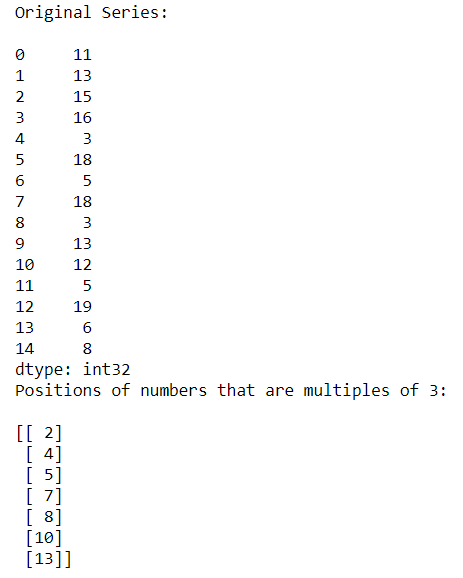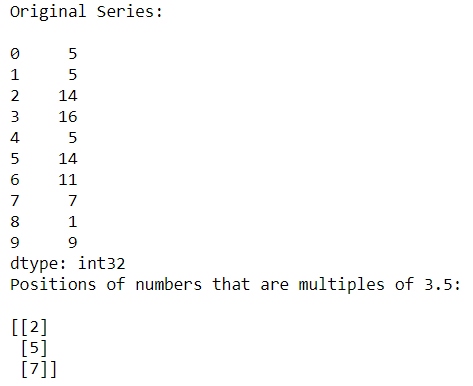# Find the position of number that is multiple of certain number

• Last Updated : 11 Oct, 2020

In this type of question, a number is given and we have to find the position or index of all multiples of that number. For doing this question we use a function named numpy.argwhere().

Syntax:

Attention geek! Strengthen your foundations with the Python Programming Foundation Course and learn the basics.

To begin with, your interview preparations Enhance your Data Structures concepts with the Python DS Course. And to begin with your Machine Learning Journey, join the Machine Learning - Basic Level Course

```numpy.argwhere(array)
```

Example 1:

Sometimes we need to find the indexes of the element that are divisible by int or float number.

## Python3

 `# Importing Pandas and Numpy libraries``import` `pandas as pd``import` `numpy as np`` ` `# Creating a Series of random numbers``n_series ``=` `pd.Series(np.random.randint(``1``, ``25``, ``15``))``print``(``"Original Series:\n"``)``print``(n_series)`` ` `# Finding the indexes of numbers divisible by 3``res_index ``=` `np.argwhere(n_series ``%` `3``=``=``0``)``print``(``"Positions of numbers that are multiples of 3:\n"``)``print``(res_index)`

Output:In the above example, we find the indexes of all numbers divisible by 3.

Example 2:

## Python3

 `# Importing Pandas and Numpy libraries``import` `pandas as pd``import` `numpy as np`` ` `# Creating a Series of random numbers``n_series ``=` `pd.Series(np.random.randint(``1``, ``20``, ``10``))``print``(``"Original Series:\n"``)``print``(n_series)`` ` `# Finding the indexes of numbers divisible by 3.5``res_index ``=` `np.argwhere(n_series ``%` `3.5``=``=``0``)``print``(``"Positions of numbers that are multiples of 3.5:\n"``)``print``(res_index)`

Output:In the above example, we find the indexes of all numbers divisible by floating-point number 3.5

My Personal Notes arrow_drop_up